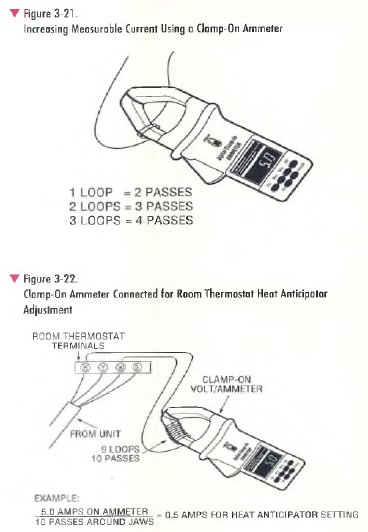Ammeters measure current flow through a circuit. Depending on the level of the current being measured, the ammeter reads in amperes, milliamperes (one thousandth of an ampere) or microamperes (one millionth of an ampere). Current must flow directly through the ammeter meter movement for it to record. For this reason, standard (in-line) ammeters such as those incorporated in multimeters, must always be connected in series with the load. This requires disconnecting or opening the normal circuit to insert the ammeter. Because of this inconvenience, as well as for safety reasons, the A C clamp-on ammeter is used almost exclusively for HVAC field service.

The clamp-on ammeter is most often used to measure the total AC current being drawn by a system or by individual loads like the compressor, fan motors, or heaters (Figure 3-20). For servicing three-phase systems, it is used to measure the current drawn by each phase to determine the percent of current imbalance* if any.

Clamp-on ammeters have a movable set o f jaws that can be opened and placed (clamped) around each of the wires to be measured, one wire at a time. The clamp-on ammeter works like a transformer. The wire being measured acts like the primary of a transformer and the “jaws” of the ammeter as the secondary. Current flowing through the wire creates lines of force that induce a current in the jaws. The induced current passes through the meter movement, providing an indication of how much current is passing through the wire. A clamp-on ammeter is easy to use, but a review of a few basic techniques will help you to obtain maximum performance.

• The jaws must be clean and properly aligned, or an error in the reading can result.
• Always start to measure within the highest possible measurement range and work toward the lower range to prevent
damage to the meter.
• Do not turn a motor off and then on with the meter clamped around the motor lead. This precaution prevents damage to the meter from current surges.
• Do not clamp the jaws of the meter around two different wires at the same time. This will cause the meter to read an incorrect value of current.

Sometimes, the current being measured will be so low that it is difficult to get an accurate measurement, even on the lowest scale of the meter. One way to overcome this problem is to coil the wire through the jaws of the meter (Figure 3-21).Winding one loop of the wire (two passes) around the jaws doubles the strength of the magnetic field, resulting in a meter reading that is twice the amount of current than is actually flowing in the circuit. If one loop is passed through the jaws, you must divide the meter reading by two (because of two passes) to determine the actual current. If two loops are passed through the jaws, you divide by three (three passes), and so on.

The adjustment of a room thermostat heat anticipator is a practical example of a procedure where this method is commonly used. The thermostat heat anticipator must be set to match the current draw of the components (heat control, gas valve, etc.) connected in the R-W circuit. As shown in Figure 3-22, an insulated wire is looped nine times around the jaws (ten passes through the jaws) of a clamp-on ammeter, then connected to the R and W terminals of the thermostat. For the purpose of this example, assume that the ammeter reads 5 amperes. Based on this measurement, you would adjust the heat anticipator to 0.5 amps (5 amps h- 10 passes = 0.5 amps).